# Visualization of Support Vector Machine¶

## Created by: Zhao Lian, Ruimeng Yang, Jingyi Liu¶

The problem below is part of an exercise problem taken from the book: "Optimization - Theory and Practice" by Wilhelm Forst and Deiter Hoffmann, Springer New York, 2010. Chapter 2, Exercise 20.

Question: Calculate a support vector 'machine' for breast cancer diagnosis using the file wisconsin-breast-cancer.data from the Breast Cancer Wisconsin Data Set (cf. http://archive.ics.uci.edu/ml/). The file wisconsin-breast- cancer.names gives information on the data set: It contains 699 instances consisting of 11 attributes. The first attribute gives the sample code number. Attributes 2 through 10 describe the medical status and give a 9-dimensional vector xi. The last attribute is the class attribute ("2" for benign, "4" for malignant). Sixteen samples have a missing attribute, denoted by “?”. Remove these samples from the data set. Now split the data into two portions: The first 120 instances are used as training data. Take software of your choice to solve the quadratic problem (P), using the penalty parameter C = 1000. The remaining instances are used to evaluate the 'performance' of the classifier or decision function given by f(x) := sgn{<w,x> + β}.

In :
import numpy as np
import pandas as pd
import matplotlib.pyplot as plt
import pylab as pl
import cvxopt
import cvxopt.solvers
from sklearn.decomposition import PCA
from sklearn import svm
from mpl_toolkits.mplot3d import Axes3D
import seaborn as sns

In :
##DEFINE THE SVM AND USE cvxopt TO SOLEVE QUADRATIC PROBLEM
class SVM(object):

#define the kernel
def linear_kernel(x1, x2):
return np.dot(x1, x2)

def __init__(self, kernel=linear_kernel, C=None):
self.kernel = kernel
self.C = C
if self.C is not None:
self.C = float(self.C)

def fit(self, X, y):
n_samples, n_features = X.shape

K = np.zeros((n_samples, n_samples))
for i in range(n_samples):
for j in range(n_samples):
K[i,j] = self.kernel(X[i], X[j])

P = cvxopt.matrix(np.outer(y,y) * K)
q = cvxopt.matrix(np.ones(n_samples) * -1)
A = cvxopt.matrix(y, (1,n_samples),'d')
b = cvxopt.matrix(0.0)

tP1 = np.diag(np.ones(n_samples) * -1)
tP2 = np.identity(n_samples)
G = cvxopt.matrix(np.vstack((tP1, tP2)))
tP1 = np.zeros(n_samples)
tP2 = np.ones(n_samples) * self.C
h = cvxopt.matrix(np.hstack((tP1, tP2)))

# solve QP problem
solution = cvxopt.solvers.qp(P, q, G, h, A, b)
cvxopt.solvers.options['show_progress'] = False

a = np.ravel(solution['x'])

# Support vectors have nonzero lamnda
sv = a > 1e-3
ind = np.arange(len(a))[sv]
self.a = a[sv]
self.sv = X[sv]
self.sv_y = y[sv]
print("%d support vectors out of %d points" % (len(self.a), n_samples))
print("The support vectors are:",self.sv,sep='\n')

# Intercept
self.b = 0
for n in range(len(self.a)):
self.b += self.sv_y[n]
self.b -= np.sum(self.a * self.sv_y * K[ind[n],sv])
self.b /= len(self.a)

# Weight vector
self.w = np.zeros(n_features)
for n in range(len(self.a)):
self.w += self.a[n] * self.sv_y[n] * self.sv[n]

def project(self, X):
return np.dot(X, self.w) + self.b

def predict(self, X):
return np.sign(self.project(X))

def support_vectors(self, X):
return self.sv

##DEFINE THE TEST OF SOFT MARGIN
def test_soft(X_train, Y_train,X_test, Y_test):
clf = SVM(C=1000)
clf.fit(X_train, Y_train)

y_predict = clf.predict(X_test)
correct = np.sum(y_predict == Y_test)
test = np.where(y_predict == Y_test,y_predict,0)
accuracy = correct/(len(y_predict))
#print("\n\nCompare differences between the test data and the solution through the SVM:",test,sep='\n' )
print("%d out of %d predictions correct" % (correct, len(y_predict)))
print("The accuracy of the SVM is:",accuracy)

##DATA IMPORT & CLEANING
BCW.drop(['ID'], 1, inplace = True)
BCW_data = np.array(BCW.drop(['Class'],1))
BCW_result = np.array(BCW['Class'])

#For 3D
pca3 = PCA(n_components=3).fit(BCW_data)
BCW_3d = pca3.transform(BCW_data)
#separate into training and testing
X_train3 = BCW_3d[:120]
X_test3 = BCW_3d[120:]

#For 2D
pca2 = PCA(n_components=2).fit(BCW_data)
BCW_2d = pca2.transform(BCW_data)
#separate into training and testing
X_train2 = BCW_2d[:120]
X_test2 = BCW_2d[120:]

# separate into training and testing
Y_train = BCW_result[:120]
Y_test = BCW_result[120:]

print("The result of 2D:")
test_soft(X_train2, Y_train, X_test2, Y_test)
print("\nThe result of 3D:")
test_soft(X_train3, Y_train, X_test3, Y_test)

The result of 2D:
pcost       dcost       gap    pres   dres
0: -4.2477e+03 -6.8559e+07  2e+08  6e-01  2e-11
1:  1.3909e+04 -2.1351e+07  4e+07  1e-01  2e-11
2:  1.3222e+04 -3.7700e+06  5e+06  1e-02  2e-11
3: -5.6541e+03 -3.9252e+05  4e+05  3e-04  2e-11
4: -6.3255e+03 -2.2280e+04  2e+04  1e-05  3e-11
5: -7.8006e+03 -1.8833e+04  1e+04  7e-06  2e-11
6: -6.6526e+03 -1.7428e+04  1e+04  6e-06  2e-11
7: -7.7576e+03 -1.8509e+04  1e+04  6e-06  2e-11
8: -8.4187e+03 -1.5954e+04  8e+03  3e-06  2e-11
9: -8.9084e+03 -1.5076e+04  6e+03  2e-06  2e-11
10: -9.2168e+03 -1.3695e+04  4e+03  1e-06  2e-11
11: -9.2066e+03 -1.2788e+04  4e+03  8e-07  2e-11
12: -9.8434e+03 -1.2076e+04  2e+03  1e-07  2e-11
13: -1.0200e+04 -1.1390e+04  1e+03  7e-08  2e-11
14: -1.0183e+04 -1.1174e+04  1e+03  3e-08  2e-11
15: -1.0231e+04 -1.1102e+04  9e+02  2e-08  2e-11
16: -1.0521e+04 -1.0680e+04  2e+02  9e-10  3e-11
17: -1.0558e+04 -1.0634e+04  8e+01  2e-10  3e-11
18: -1.0588e+04 -1.0598e+04  1e+01  2e-11  3e-11
19: -1.0593e+04 -1.0593e+04  1e-01  7e-13  3e-11
20: -1.0593e+04 -1.0593e+04  1e-03  1e-12  3e-11
Optimal solution found.
13 support vectors out of 120 points
The support vectors are:
[[ 4.81965073 -4.73973426]
[ 5.0965347   3.38659877]
[-1.68552372 -6.70707678]
[ 0.0146741   0.47181194]
[-2.86282194  0.01414944]
[ 0.49641139 -0.41543497]
[ 0.76106488  3.11693006]
[ 2.2740231  -5.62395287]
[-2.22153501  1.5122255 ]
[ 0.50403378 -0.3500689 ]
[ 0.65009279 -0.3635259 ]
[-2.66948305  1.04255784]
[-1.20128661  0.28138796]]
547 out of 563 predictions correct
The accuracy of the SVM is: 0.9715808170515098

The result of 3D:
13 support vectors out of 120 points
The support vectors are:
[[ 4.81965073 -4.73973426  1.0075824 ]
[ 5.0965347   3.38659877 -2.16129027]
[-1.68552372 -6.70707678  2.53036905]
[ 0.0146741   0.47181194 -0.26175299]
[-2.86282194  0.01414944 -2.13872282]
[ 0.49641139 -0.41543497  0.18311174]
[ 0.76106488  3.11693006 -3.70324074]
[ 2.2740231  -5.62395287 -0.68140337]
[-2.22153501  1.5122255   2.58537644]
[ 0.50403378 -0.3500689   2.76941413]
[ 0.65009279 -0.3635259  -3.64653468]
[-2.66948305  1.04255784  2.74323475]
[-1.20128661  0.28138796 -4.24877478]]
548 out of 563 predictions correct
The accuracy of the SVM is: 0.9733570159857904


# Result¶

Based on the results above, after using PCA method to reduce the dminsion from nine to two, 547 out of 563 samples in the testing set are correctly predicted. And 548 out of 563 samples in the testing set are correctly predicted after using PCA method to reduce the dminsion to three. Under both situation, the accuracy of the SVM is higher than 97%.

The same result will be given using the original nine dimension data, but in order to visualize SVM, we use a dimension-reduction tool, Principle Component Analysis, to reduce the large set of variables to a small set that still contains most of the information in the large set.

In :
#hyperplane
clf = svm.SVC(kernel='linear', C=1000)
clf.fit(X_train2, Y_train)

# plt.scatter(X_train2[:, 0], X_train2[:, 1], c=Y_train, s=50, cmap=plt.cm.Paired)

for i in range(0, 120):
if Y_train[i] == 1:
d1=plt.scatter(X_train2[i,0], X_train2[i,1],  c='r', marker='o')
else:
d2=plt.scatter(X_train2[i,0], X_train2[i,1],  c='g', marker='o')

plt.legend([d1,d2],['benign','malignant'],loc='upper left')

# plot the decision function
ax = plt.gca()
xlim = ax.get_xlim()
ylim = ax.get_ylim()

# create grid to evaluate model
xx=np.linspace(xlim, xlim, 100)
yy=np.linspace(ylim, ylim, 100)
YY, XX = np.meshgrid(yy, xx)
xy = np.vstack([XX.ravel(), YY.ravel()]).T
Z = clf.decision_function(xy).reshape(XX.shape)

# plot decision boundary and margins
ax.contour(XX, YY, Z, colors='k', levels=[-1, 0, 1], alpha=0.5, linestyles=['--', '-', '--'])

# plot support vectors
ax.scatter(clf.support_vectors_[:, 0], clf.support_vectors_[:, 1], s=60,
linewidth=1, facecolors='none', edgecolors='k')

plt.show()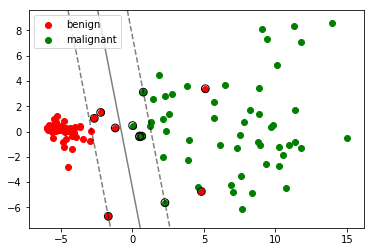For the 2D situation, we can see that the hyperplane separates the training data into two classes and all the support vectors are marked by the black circle.

When we tint the data points with different color according to the reality. It is clearly that there are two outliers for the benign class. But for the rest of the data points, SVM shows a very good result.

In :
fig = plt.figure()
ax = fig.gca(projection='3d')

#Training
cls = svm.SVC(kernel='linear', C=1000)
cls.fit(X_train3, Y_train)

w = cls.coef_
b = cls.intercept_

#Plot Hyperplane
ax = plt.subplot(111, projection='3d')
x = np.arange(-10,10,0.01)
y = np.arange(-10,10,0.11)
x, y = np.meshgrid(x, y)
z = (w[0,0]*x + w[0,1]*y + b) / (-w[0,2])
surf = ax.plot_surface(x, y, z)

# Plot scatter
x_array = np.array(X_train3, dtype=float)
y_array = np.array(Y_train, dtype=int)
be = x_array[np.where(y_array==1)]
ma = x_array[np.where(y_array==-1)]
ax.scatter(be[:,0], be[:,1], be[:,2], c='r', label='benign')
ax.scatter(ma[:,0], ma[:,1], ma[:,2], c='g', label='malignant')

# Plot support vectors
X = np.array(X_train3,dtype=float)
for i in range(len(sv_idx)):
ax.scatter(X[sv_idx[i],0], X[sv_idx[i],1], X[sv_idx[i],2],s=30,
c='',marker='o', edgecolors='k')

# Set lables
ax.set_zlabel('Z')
ax.set_ylabel('Y')
ax.set_xlabel('X')
ax.set_zlim([-90, 90])
plt.legend(loc='upper left')

plt.show()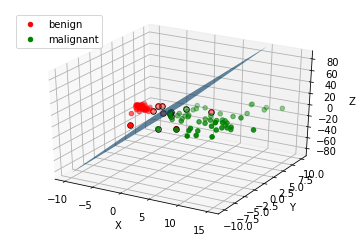The hyperplane in the 3D shows a pretty much same result with the 2D plot.

In :
#Plot the heat map to see the correlation among attributes.
sns.set(style='ticks', color_codes=True)
plt.figure(figsize=(14, 12))
sns.heatmap(BCW.astype(float).corr(),
linewidths=0.1,
square=True,
linecolor='white',
annot=True)
plt.show()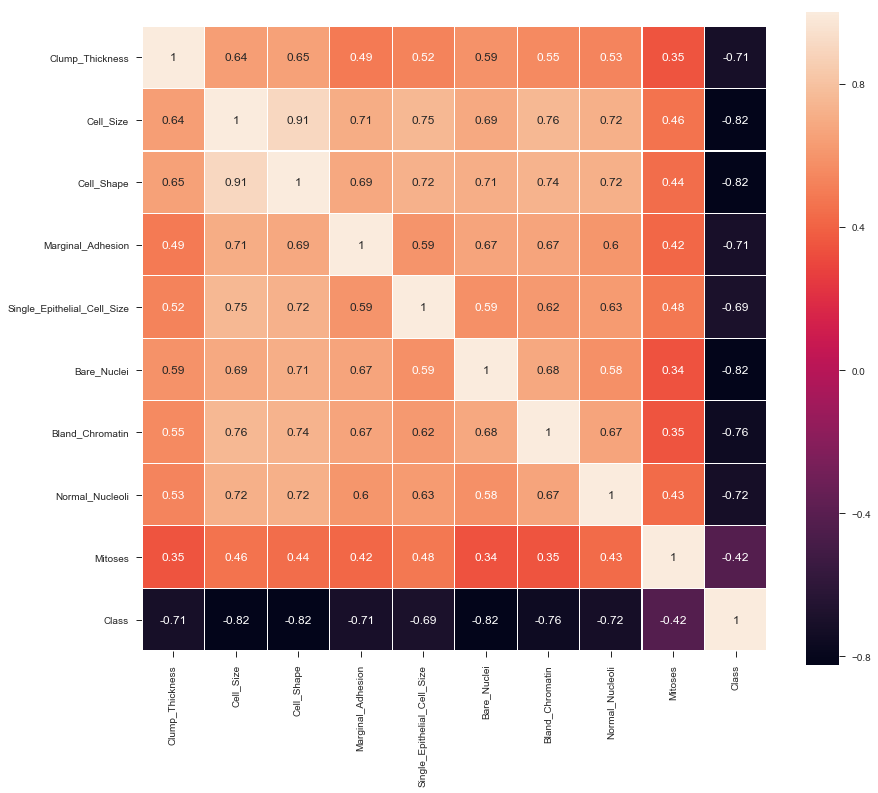The heat map shows the correlation among attributes. For example, the correlation between clump thickness and uniformity of cell size is 0.64.

In :
#Bar plot 1
fig = plt.figure()
ax = sns.countplot(x="Bland_Chromatin",
hue='Class',
#palette={0:'#EB434A', 1:'#61A98F'},
data=BCW)
ax.set(xlabel='Bland Chromatin', ylabel='No of cases')
fig.suptitle("Bland Chromatin w.r.t. Class", y=0.96);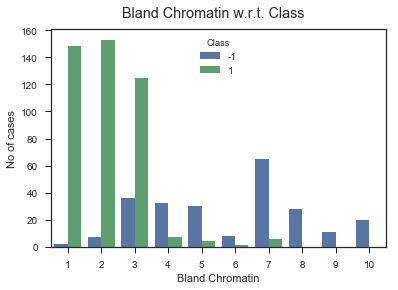This graph shows how many people of each category in bland chromation falls into class 1 or class 0. Class 1 means the beginning stage of cancer and class 0 means malignant. From the graph we can see that when bland chromatin is in the range 1 to 3, most patients are in the early stage of cancer. And if it falls into category 8 - 10, all the patient are in the malignant stage.

In :
#Bar plot 2
fig = plt.figure()
ax = sns.countplot(x='Clump_Thickness',
hue='Class',
#palette={0:'#EB434A', 1:'#61A98F'},
data=BCW)
ax.set(xlabel='Thickness of Clump', ylabel='Total')
fig.suptitle("Clump_Thickness w.r.t. Class", y=0.96);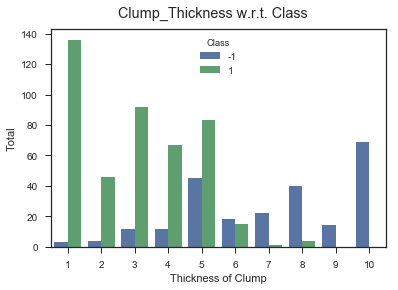Similarly, this graph shows how many people of each category in clump thickness falls into class 1 or class 0.

1. Mathieu Blondel, September 2010, http://www.mblondel.org/journal/2010/09/19/support-vector-machines-in-python/
2. Vanderplas, Jacob T. Python Data Science Handbook: Tools and Techniques for Developers. O'Reilly, 2016.